# Specific requirements

In this programming project, complete the following steps:
• Select a data set and load it into R/SPSS.
• Fit logistic regression models to analyze the data of your own choosing.
• Submit a *.html file generated by R Markdown.

# Data frame information

Data set name:
Credit Card Default Data
Description:
A simulated data set containing information on ten thousand customers. The aim here is to predict which customers will default on their credit card debt.
Usage:
Default
Format:
A data frame with 10000 observations on the following 4 variables.
default
A factor with levels No and Yes indicating whether the customer defaulted on their debt.
balance
The average balance that the customer has remaining on their credit card after making their monthly payment.
income
Income of customer.
Source:
Simulated data
References:
James, G., Witten, D., Hastie, T., and Tibshirani, R. (2013) An Introduction to Statistical Learning with applications in R, http://www.StatLearning.com Springer-Verlag, New York
Examples:
summary(Default)
glm(default~student+balance+income,family=“binomial”,data=Default)
http://vincentarelbundock.github.io/Rdatasets/datasets.html

# Include the knitr package for integration of R code into Markdown

knitr::opts_chunk$set(echo = TRUE) # Import data library(readr) input_data <- read_csv("Default.csv", col_types = cols(X1 = col_skip(), balance = col_number(), default = col_character(), income = col_number(), student = col_character())) ## Warning: Missing column names filled in: 'X1'  # Descriptive statistics ## Dimension of data frame dim(input_data) ##  10000 4 ## Structure of data frame str(input_data) ## tibble [10,000 x 4] (S3: spec_tbl_df/tbl_df/tbl/data.frame) ##$ default: chr [1:10000] "No" "No" "No" "No" ...
##  $student: chr [1:10000] "No" "Yes" "No" "No" ... ##$ balance: num [1:10000] 730 817 1074 529 786 ...
##  835.3749
##
##  823.637
##
## $income ##  34552.64 ## Mode of each variable require(modeest) ## Loading required package: modeest lapply(input_data[,3],mfv) ##$balance
##  0
##  0
##
##  2654.323
##
## $income ##  73554.23 ## Range of each variable lapply(input_data[,c(3,4)],range) ##$balance
##     0.000 2654.323
##
##  233980.2
##
##  483.715
##
## $income ##  13336.64 ## Median absolute deviation of each variable lapply(input_data[,c(3,4)],mad) ##$balance
##  507.5173
##
## $income ##  16350.86 # Plots and Graphs ## Box plots ### Explanation: These box plots reveal the mean value, minimum value, and maximum value of each variable. ### Observation: Customers with higher average income are less likely to default on their credit cards. Customers with lower average balance are less likely to default on their credit cards. Students have higher average balances than non-student customers. Students have lower average incomes than non-student customers. library(ggplot2) ggplot(data = input_data, aes(x=, y=balance, fill=default)) + geom_boxplot(outlier.colour = "red") + xlab("") + ylab("Balance") + ggtitle("Balance by Default Status")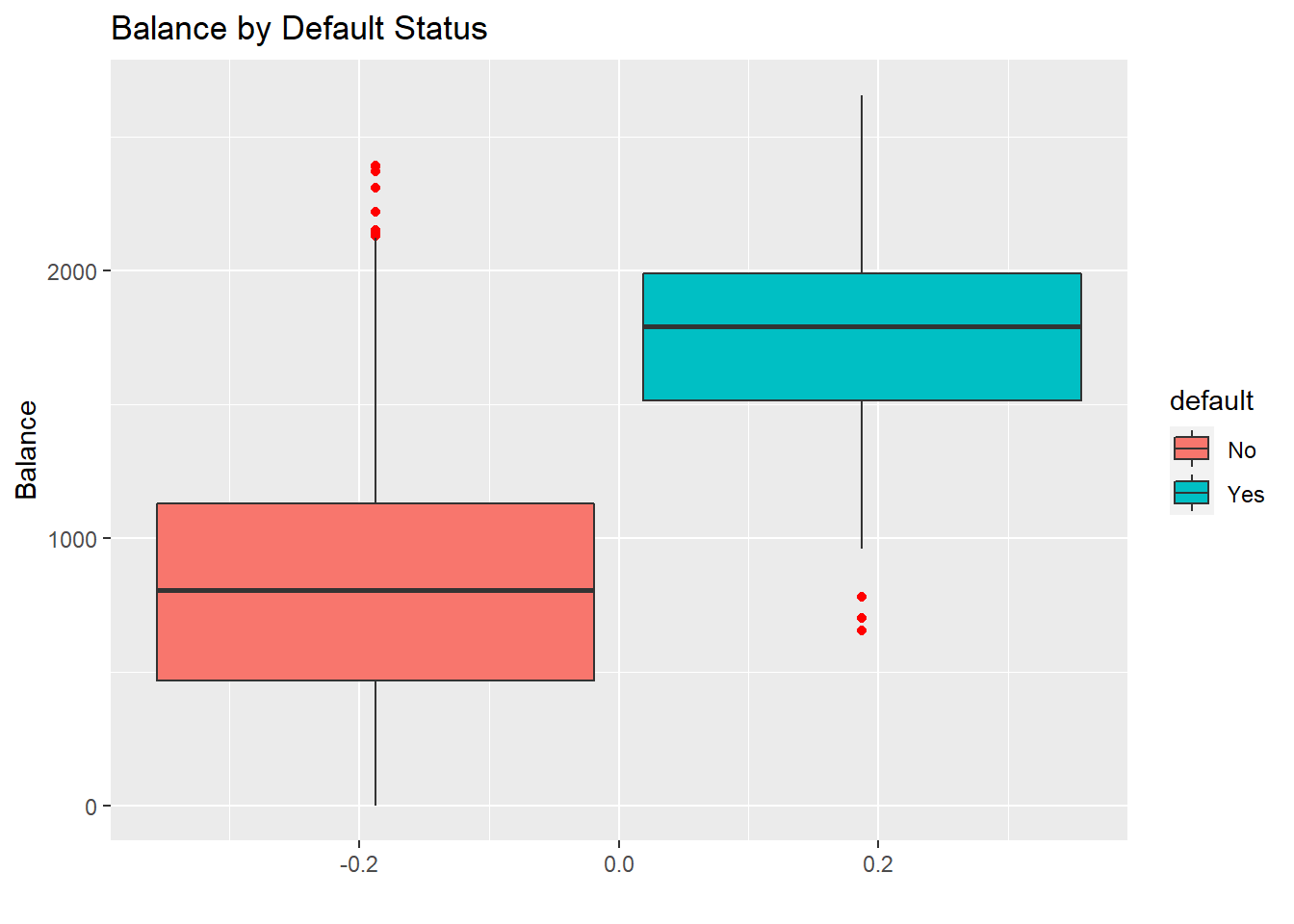library(ggplot2) ggplot(data = input_data, aes(x=, y=income, fill=default)) + geom_boxplot(outlier.colour = "red") + xlab("") + ylab("Income") + ggtitle("Income by Default Status")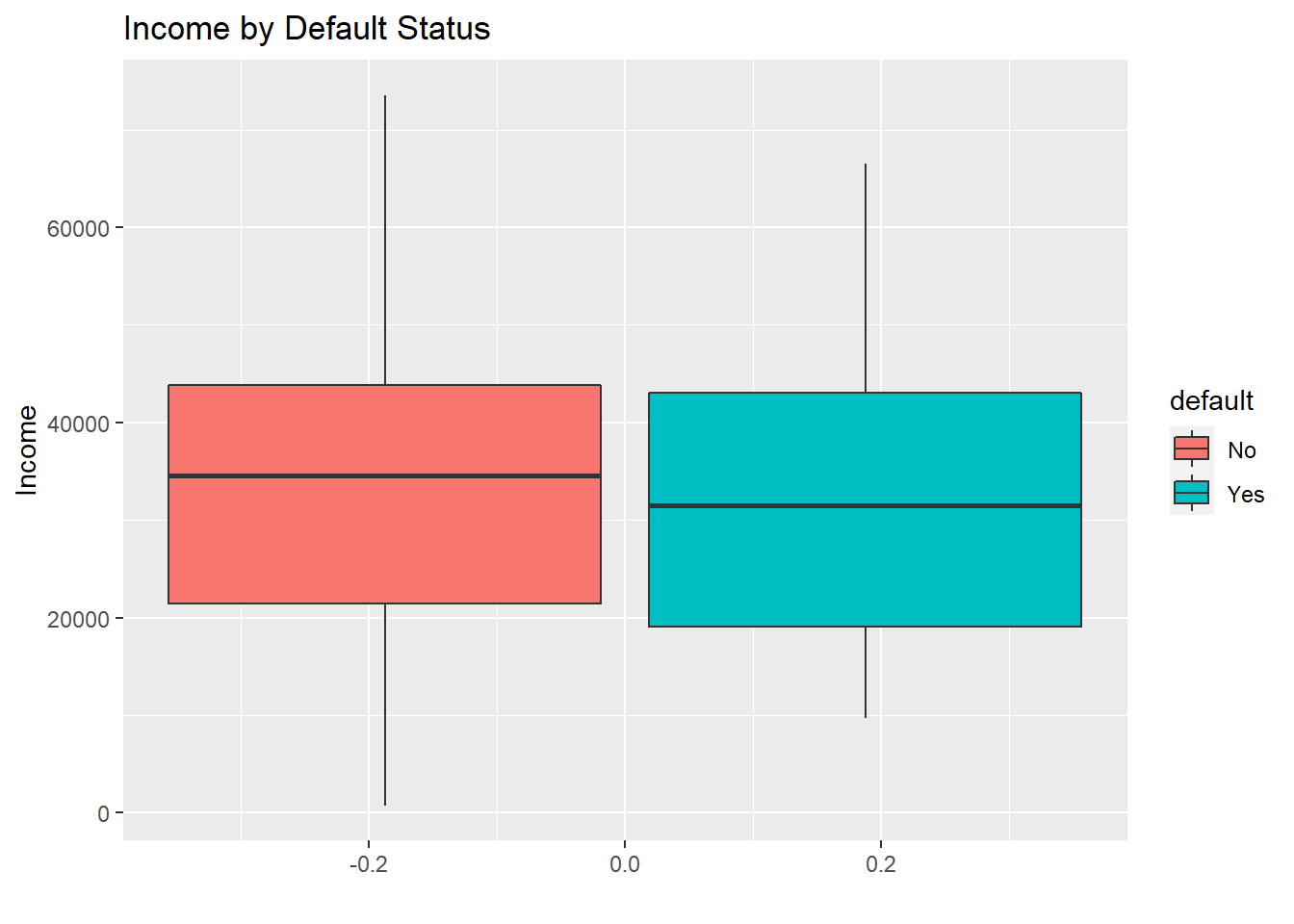ggplot(data = input_data, aes(x=, y=balance, fill=student)) + geom_boxplot(outlier.colour = "red") + xlab("") + ylab("Balance") + ggtitle("Balance by Student Status")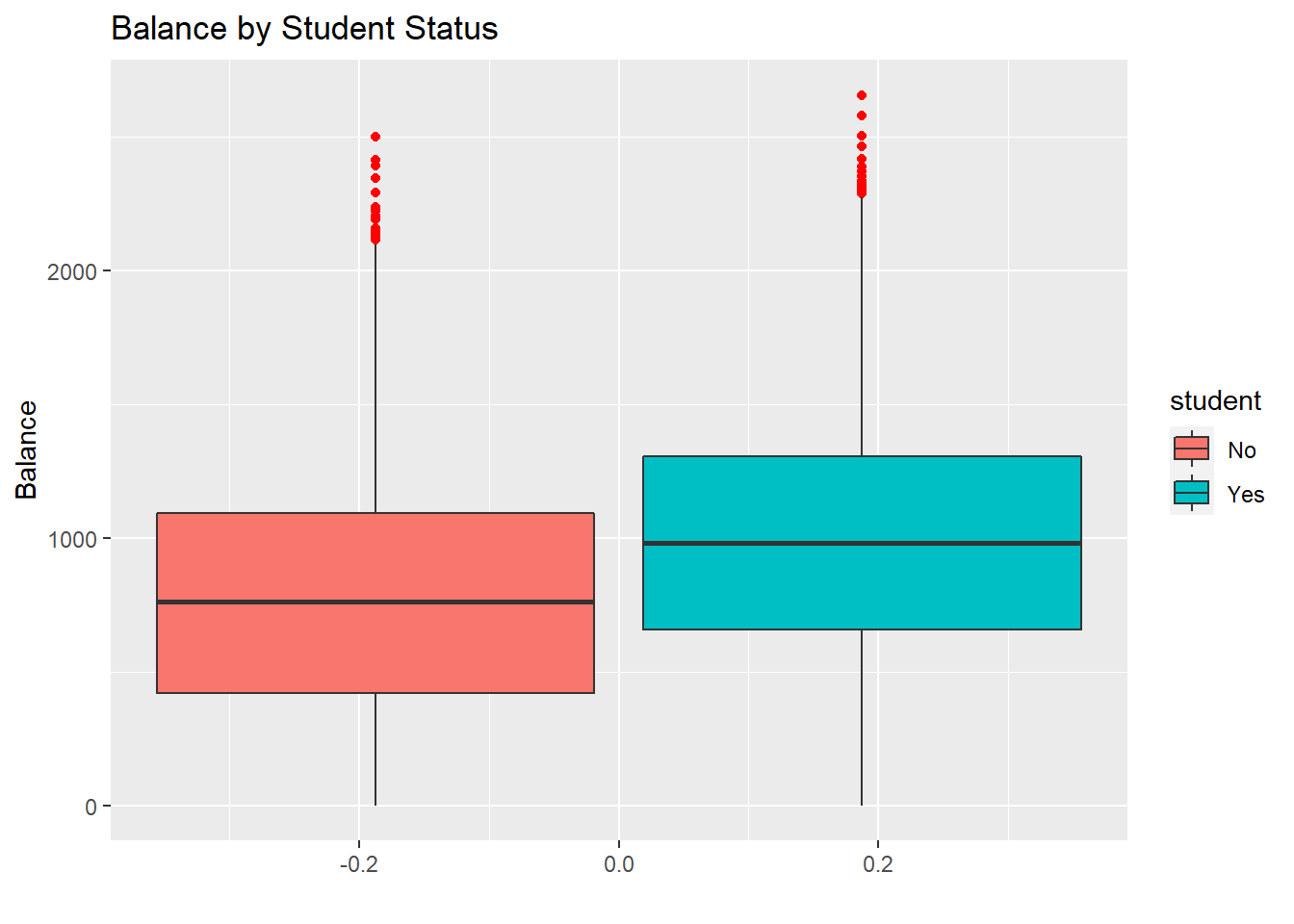library(ggplot2) ggplot(data = input_data, aes(x=, y=income, fill=student)) + geom_boxplot(outlier.colour = "red") + xlab("") + ylab("Income") + ggtitle("Income by Student Status")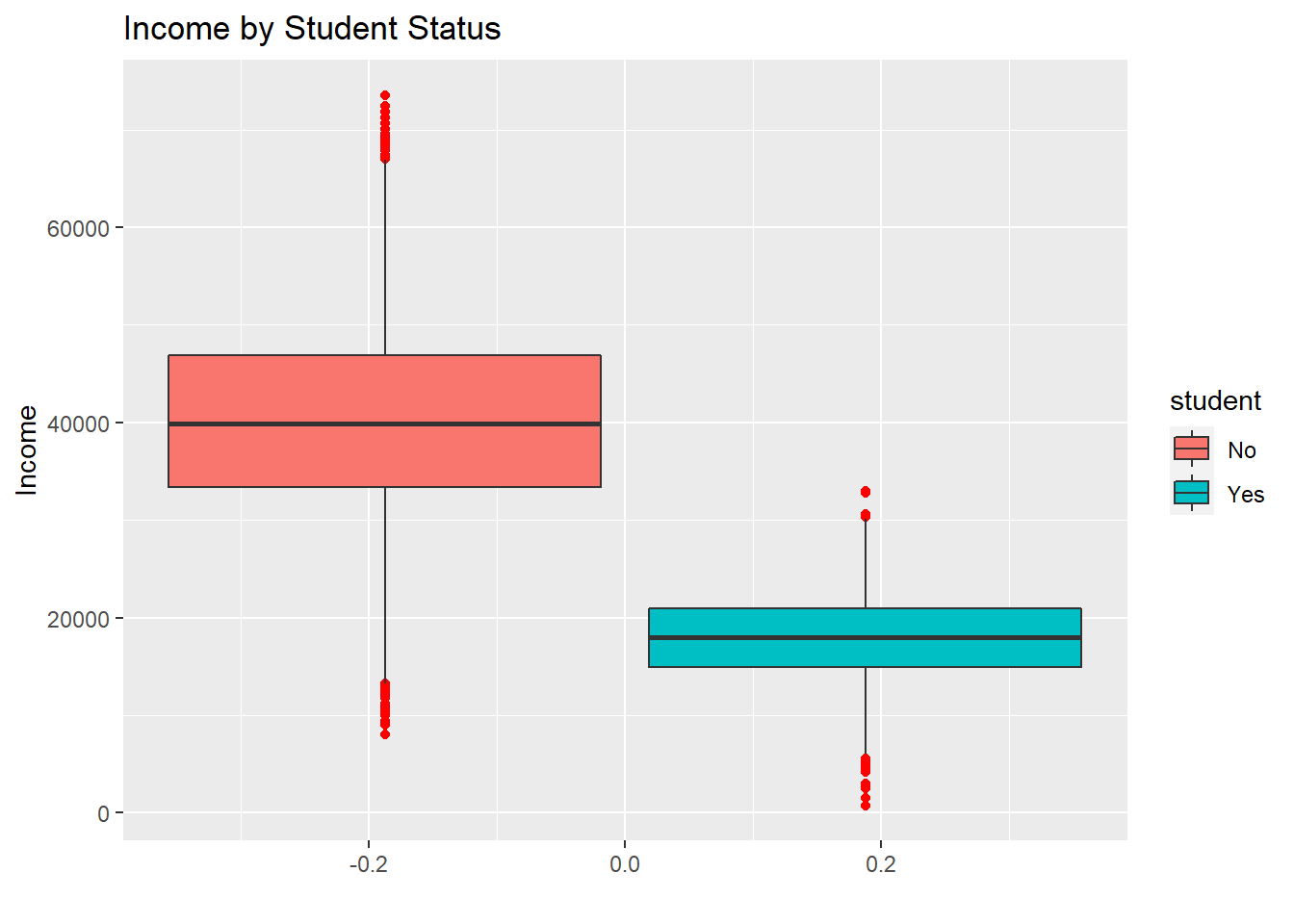# Histograms ### Explanation: These histogram plots reveal the relationships between customer student status, default status, income and balance. ### Observation: Majority of customers who default on their credit cards have balance of about$1,800. Majority of customers who default on their credit cards have income of about $19,000. Majority of the customers are non-students. Students have much lower balances than non-student customers. Students have much lower incomes than non-student customers. library(ggplot2) ggplot(data = input_data, aes(x=balance, fill=default, color=default)) + geom_histogram(alpha=0.6)+ xlab("Balance") + ylab("Customer Count") + ggtitle("Balance by Default Status") ## stat_bin() using bins = 30. Pick better value with binwidth.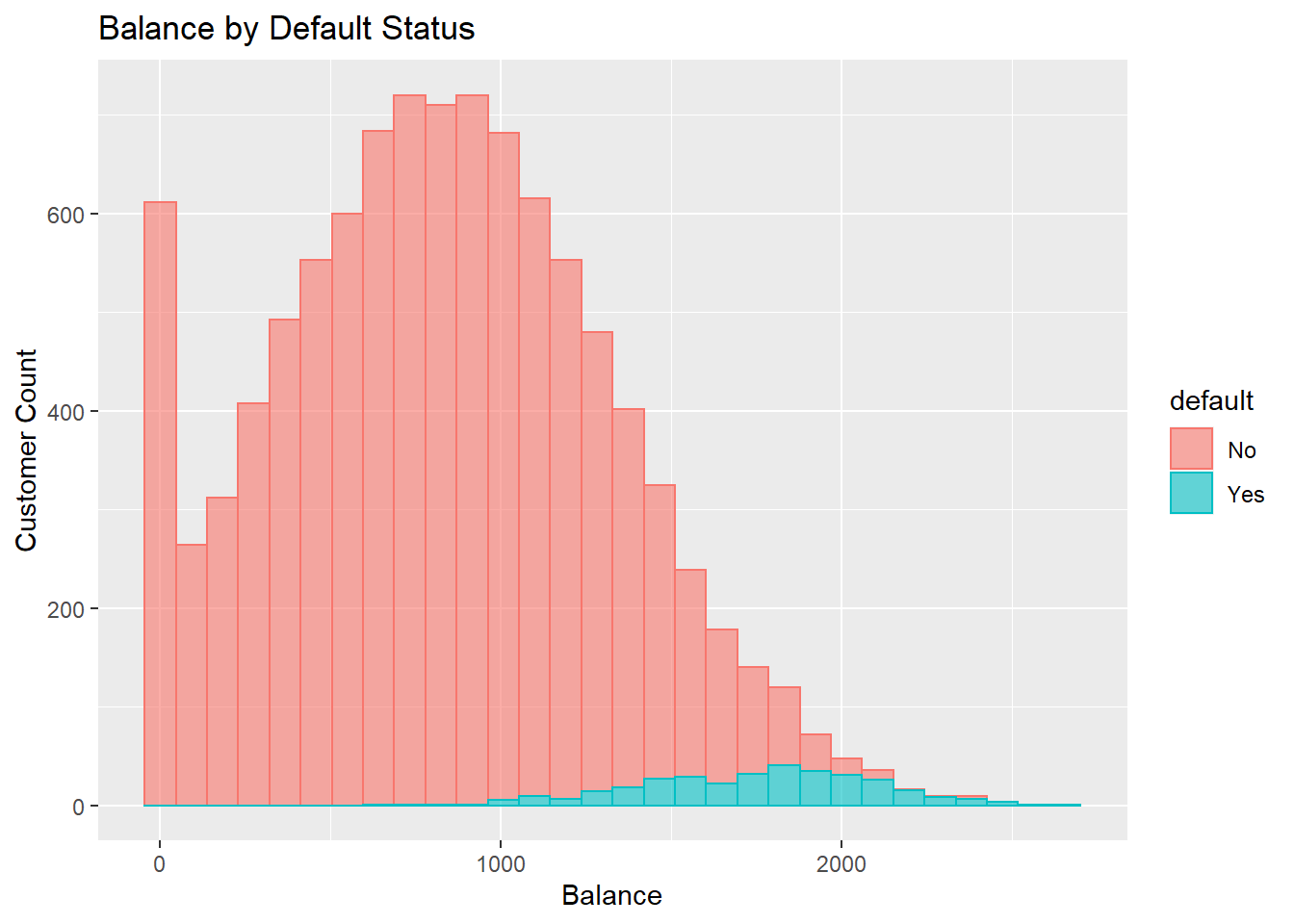ggplot(data = input_data, aes(x=income, fill=default, color=default)) + geom_histogram(alpha=0.6) + xlab("Income") + ylab("Customer Count") + ggtitle("Income by Default Status") ## stat_bin() using bins = 30. Pick better value with binwidth.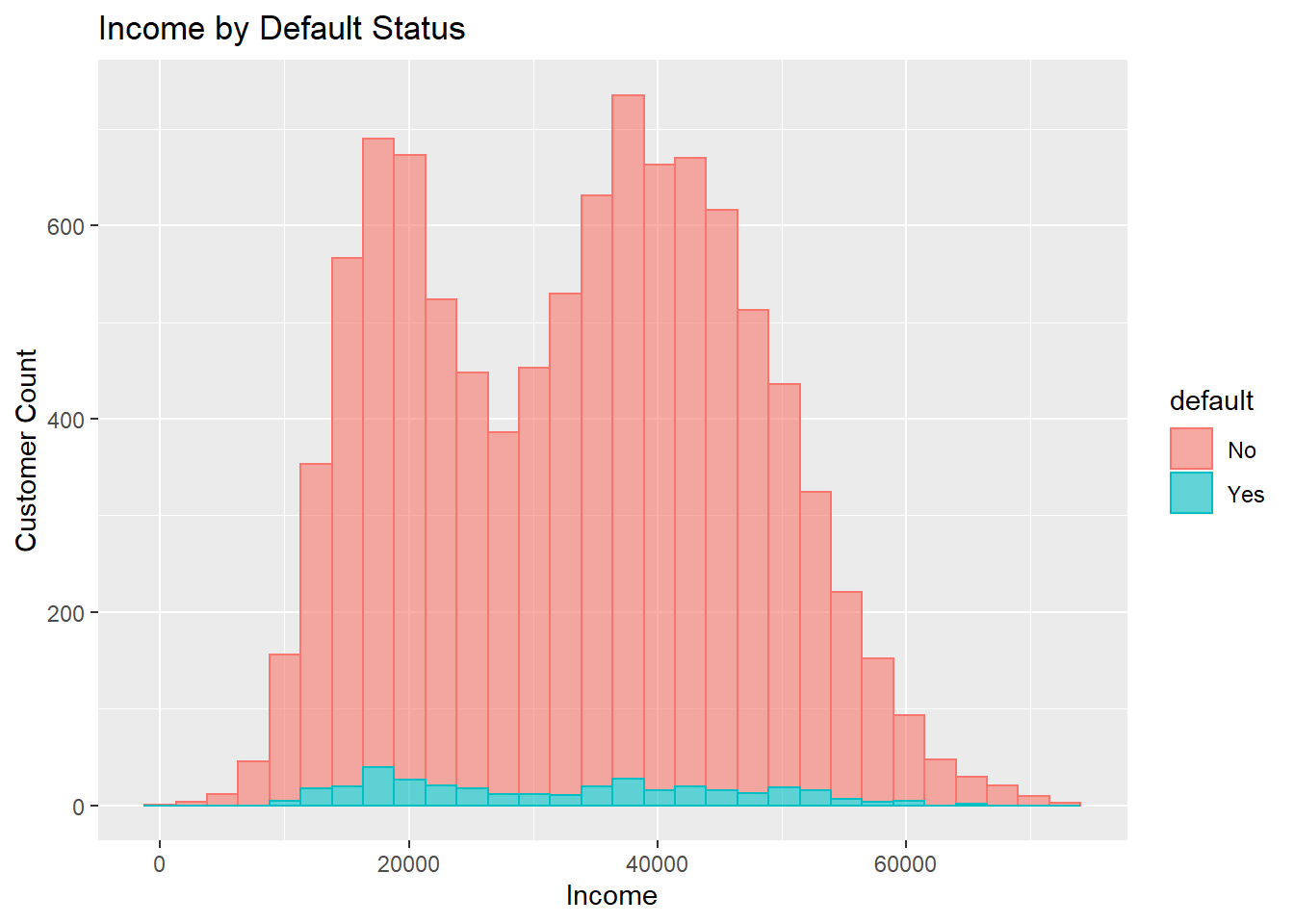ggplot(data = input_data, aes(x=balance, fill=student, color=student)) + geom_histogram(alpha=0.6) + xlab("Balance") + ylab("Customer Count") + ggtitle("Balance by Student Status") ## stat_bin() using bins = 30. Pick better value with binwidth.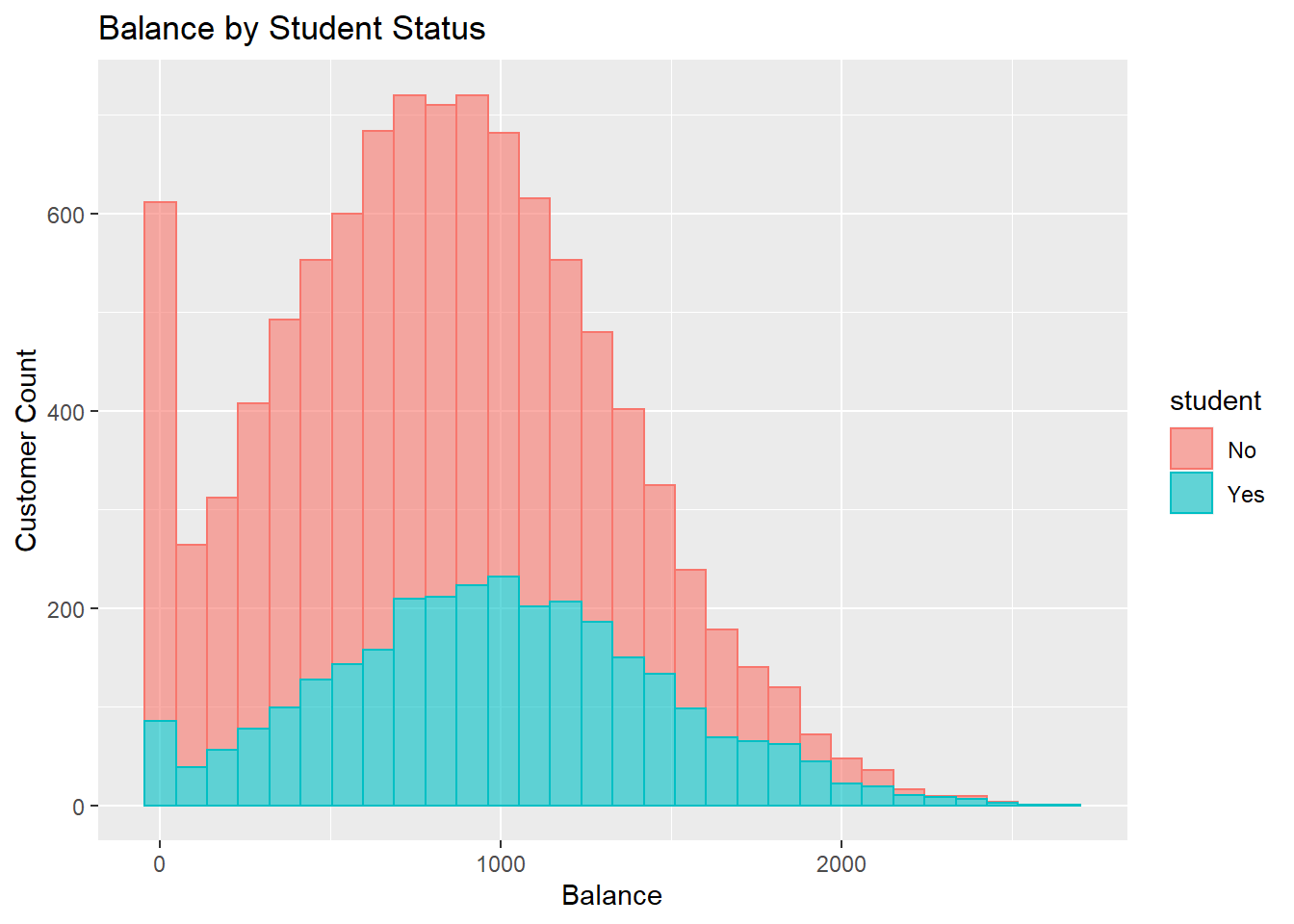ggplot(data = input_data, aes(x=income, fill=student, color=student)) + geom_histogram(alpha=0.6) + xlab("Income") + ylab("Customer Count") + ggtitle("Income by Student Status") ## stat_bin() using bins = 30. Pick better value with binwidth.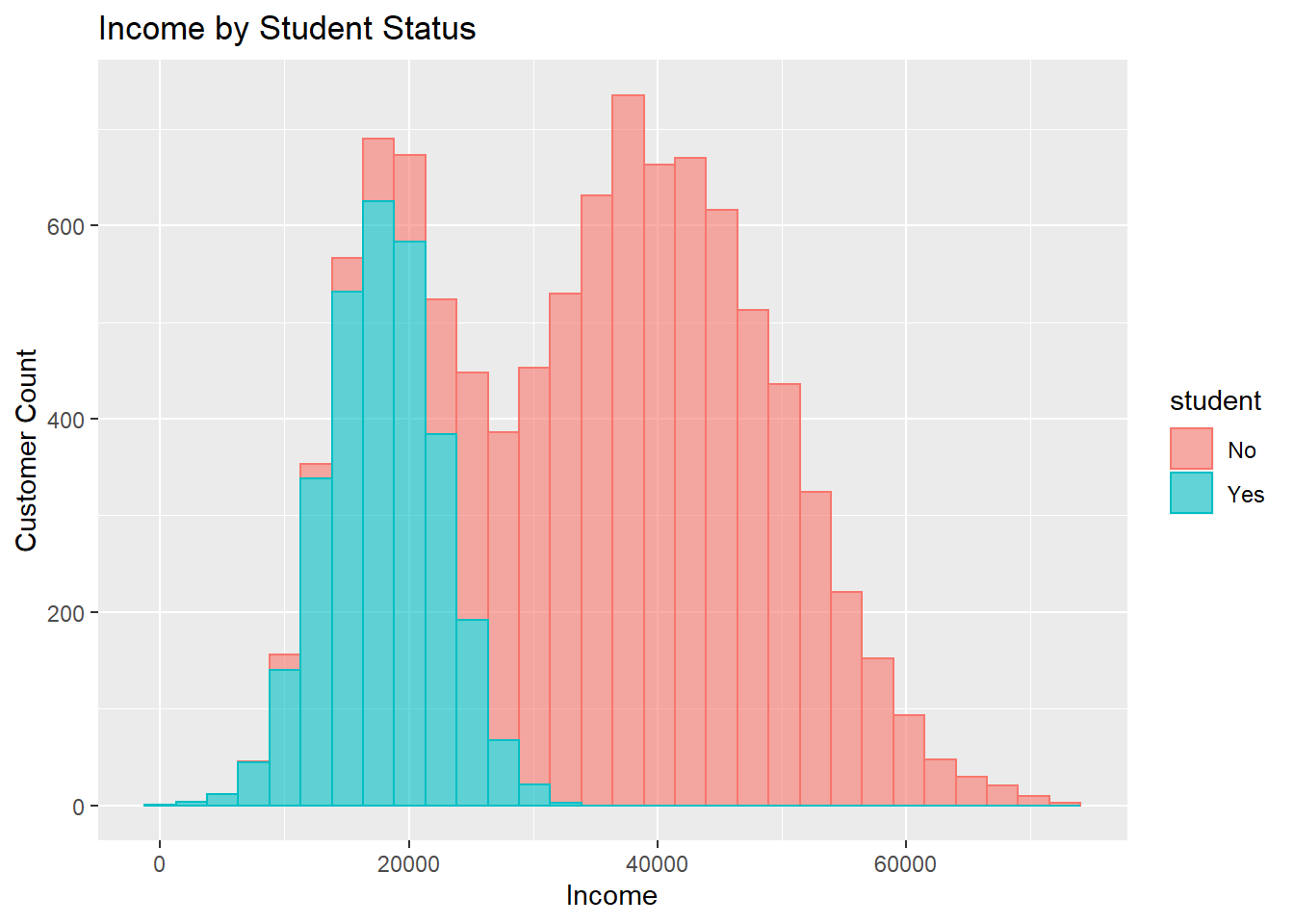# Regression Model Fitting ## Correlation Statistics ### Explanation: The correlation statistics reveal the degree of associations between variables in the data set. ### Observation: It appears that there is not much association between independent variables balance and income. A correlation coefficient value of -0.15 and a p-value of 2.2e-16 indicate a very weak correlation between the two variables. ### Coefficient R: cor(input_data[,c(3,4)]) ## balance income ## balance 1.0000000 -0.1522434 ## income -0.1522434 1.0000000 ### P-Value: library(Hmisc) ## Loading required package: lattice ## Loading required package: survival ## Loading required package: Formula ## ## Attaching package: 'Hmisc' ## The following objects are masked from 'package:base': ## ## format.pval, units attach(input_data[,c(3,4)]) cor.test(balance,income) ## ## Pearson's product-moment correlation ## ## data: balance and income ## t = -15.402, df = 9998, p-value < 2.2e-16 ## alternative hypothesis: true correlation is not equal to 0 ## 95 percent confidence interval: ## -0.1713322 -0.1330403 ## sample estimates: ## cor ## -0.1522434 ## Converting categorical variables into factors ### Explanation: Variables default and student are categorical character variables. Since in order for R’s logistic regression to work correctly, all categorical variables much be explicitly converted into factors. input_data$default = as.factor(input_data$default) input_data$student = as.factor(input_data$student) str(input_data) ## tibble [10,000 x 4] (S3: spec_tbl_df/tbl_df/tbl/data.frame) ##$ default: Factor w/ 2 levels "No","Yes": 1 1 1 1 1 1 1 1 1 1 ...
##  $student: Factor w/ 2 levels "No","Yes": 1 2 1 1 1 2 1 2 1 1 ... ##$ balance: num [1:10000] 730 817 1074 529 786 ...
##  $income : num [1:10000] 44362 12106 31767 35704 38463 ... ## - attr(*, "spec")= ## .. cols( ## .. X1 = col_skip(), ## .. default = col_character(), ## .. student = col_character(), ## .. balance = col_number(), ## .. income = col_number() ## .. ) ### Relationship between categorical variables: xtabs(~default + student, data=input_data) ## student ## default No Yes ## No 6850 2817 ## Yes 206 127 ## 1st Stepwise Regression Model Iteration ### Explanation: This is the first stepwise iteration of the regression model. This step will reveal the model statistics such as the R-squared value, the Akaike information criterion (AIC), and the P-value of the logistic regression. The R-squared value indicates the percentage of the actual data points that could be explained by the regression. The AIC value is a maximum likelihood estimator for the logistic regression model. The smaller the AIC value the better fitted the model. The p-value of each independent variable indicates the pairwise significance of the association between each independent variable and the dependent variable. ### Observation: A McFadden R-squared value of 0.46 means that the regression can explain 46% of the actual data points. Given a range between 0 and 1, an R-Squared value of 0.46 indicates a moderately fitted regression model. Given a cut-off point of 0.05, the p-value 1 of independent variable income is not significant at all. However, the p-value 0.0 of the independent variables “balance” is very significant. The p-value 0.001 of the dummy variable “studentYes” is moderately significant. Also, given a variance inflation factor cut-off point of 5, all three independent variables, student, balance, and income have very little multicollinearity. It is because they all have variance inflation factor values smaller than 3. The AIC value of 1579.5 will be compared with the AIC value in the next stepwise iteration to find the best-fitted regression model. ### Stepwise: rg_output <- glm(default~., data=input_data, family=binomial(link="logit")) summary(rg_output) ## ## Call: ## glm(formula = default ~ ., family = binomial(link = "logit"), ## data = input_data) ## ## Deviance Residuals: ## Min 1Q Median 3Q Max ## -2.4691 -0.1418 -0.0557 -0.0203 3.7383 ## ## Coefficients: ## Estimate Std. Error z value Pr(>|z|) ## (Intercept) -1.087e+01 4.923e-01 -22.080 < 2e-16 *** ## studentYes -6.468e-01 2.363e-01 -2.738 0.00619 ** ## balance 5.737e-03 2.319e-04 24.738 < 2e-16 *** ## income 3.033e-06 8.203e-06 0.370 0.71152 ## --- ## Signif. codes: 0 '***' 0.001 '**' 0.01 '*' 0.05 '.' 0.1 ' ' 1 ## ## (Dispersion parameter for binomial family taken to be 1) ## ## Null deviance: 2920.6 on 9999 degrees of freedom ## Residual deviance: 1571.5 on 9996 degrees of freedom ## AIC: 1579.5 ## ## Number of Fisher Scoring iterations: 8 ### McFadden R-Squared: #log-likelihood of the null model ll.null <- rg_output$null.deviance/-2
#log-likelihood of the fancy model
ll.proposed <- rg_output$deviance/-2 r2 <- (ll.null-ll.proposed)/ll.null print(r2) ##  0.4619194 ### Model P-Value: pv <- 1-pchisq(2*(ll.proposed-ll.null),df=(length(rg_output$coefficients)-1))
print(pv)
##  0

### Multicollinearity and Stepwise:

library(car)
## Loading required package: carData
# Variance Inflation Factors (>5?)
vif(rg_output)
##  student  balance   income
## 2.761184 1.076946 2.674562

## 2nd Stepwise Regression Model Iteration

### Explanation:

This is the second stepwise iteration of the regression model. This step will reveal the best fitted model.

### Observation:

A McFadden R-squared value of 0.46 means that the regression can explain 46% of the actual data points. Given a range between 0 and 1, an R-Squared value of 0.46 indicates a moderately fitted regression model. In order to have a better fitted regression model, it is suggested that more data records are required. 10,000 data records are not enough. Given a cut-off point of 0.05, both the p-values 0.0 of the independent variable “balance” and dummy variable “studentYes” are very significant. Given a variance inflation factor cut-off point of 5, both independent variables, balance, and student have no multicollinearity. It is because they both have variance inflation factor values close to 1. The AIC value of 1577.7 is smaller than that of the previous stepwise iteration, which means a better-fitted regression model.

### Stepwise:

rg_output = glm(default~balance+student, data=input_data, family=binomial(link="logit"))
summary(rg_output)
##
## Call:
## glm(formula = default ~ balance + student, family = binomial(link = "logit"),
##     data = input_data)
##
## Deviance Residuals:
##     Min       1Q   Median       3Q      Max
## -2.4578  -0.1422  -0.0559  -0.0203   3.7435
##
## Coefficients:
##               Estimate Std. Error z value Pr(>|z|)
## (Intercept) -1.075e+01  3.692e-01 -29.116  < 2e-16 ***
## balance      5.738e-03  2.318e-04  24.750  < 2e-16 ***
## studentYes  -7.149e-01  1.475e-01  -4.846 1.26e-06 ***
## ---
## Signif. codes:  0 '***' 0.001 '**' 0.01 '*' 0.05 '.' 0.1 ' ' 1
##
## (Dispersion parameter for binomial family taken to be 1)
##
##     Null deviance: 2920.6  on 9999  degrees of freedom
## Residual deviance: 1571.7  on 9997  degrees of freedom
## AIC: 1577.7
##
## Number of Fisher Scoring iterations: 8

#log-likelihood of the null model
ll.null <- rg_output$null.deviance/-2 #log-likelihood of the fancy model ll.proposed <- rg_output$deviance/-2
r2 <- (ll.null-ll.proposed)/ll.null
print(r2)
##  0.4618726

### Model P-Value:

pv <- 1-pchisq(2*(ll.proposed-ll.null),df=(length(rg_output\$coefficients)-1))
print(pv)
##  0

### Multicollinearity:

library(car)
# Variance Inflation Factors (>5?)
vif(rg_output)
##  balance  student
## 1.076697 1.076697

## Automatic Stepwise Testing

### Explanation:

This is the automation of stepwise iterations.

### Observation:

The resulting suggested model reveals the same statistics as that from the second manual stepwise iteration above. Therefore, It is evident that the suggested model is the most parsimonious logistic regression model for this data frame.

m_empty = glm(default~1, data=input_data, family=binomial(link="logit"))
step(m_empty, direction = "both", scope=formula(m_all))
## Start:  AIC=2922.65
## default ~ 1
##
##           Df Deviance    AIC
## + balance  1   1596.5 1600.5
## + student  1   2908.7 2912.7
## + income   1   2916.7 2920.7
## <none>         2920.7 2922.7
##
## Step:  AIC=1600.45
## default ~ balance
##
##           Df Deviance    AIC
## + student  1   1571.7 1577.7
## + income   1   1579.0 1585.0
## <none>         1596.5 1600.5
## - balance  1   2920.7 2922.7
##
## Step:  AIC=1577.68
## default ~ balance + student
##
##           Df Deviance    AIC
## <none>         1571.7 1577.7
## + income   1   1571.5 1579.5
## - student  1   1596.5 1600.5
## - balance  1   2908.7 2912.7
##
## Call:  glm(formula = default ~ balance + student, family = binomial(link = "logit"),
##     data = input_data)
##
## Coefficients:
## (Intercept)      balance   studentYes
##  -10.749496     0.005738    -0.714878
##
## Degrees of Freedom: 9999 Total (i.e. Null);  9997 Residual
## Null Deviance:       2921
## Residual Deviance: 1572  AIC: 1578

### Final Regression Equation:

Y = ln(p/(1-p)) = b0 + b1(x1) + b2(x2)
Y = -10.749496 + 0.005738(x1) - 0.714878(x2)
p = default = (e^(bo+b1(x1)+b2(x2))) / (e^(bo+b1(x1)+b2(x2))+1)
b0 = Y-intercept = -10.749496
b1 = Coefficient 1 = 0.005738
b2 = Coefficient 2 = -0.714878
x1 = balance
x2 = studentYes

### Regression Equation Interpretation:

The Y-intercept or Coefficient b0 for this equation is -10.749496. If independent variables X1 and X2 all equal to zeros, then the log-odds Y is equal to -10.749496. Coefficient b1 has a positive sign, which means that for any increase in the value of X1, there will be a corresponding increase in the value of log-odds Y. Coefficient b2 has a negative sign, which means that for any increase in the value of X2, there will be a corresponding decrease in the value of log-odds Y. If compared between b1 and b2, Coefficient b2 is the largest in magnitude. It is almost 125 times the magnitude of b1. This means if X1 is constant, an increase in X2 by 1 will correspond to a decrease in the value of log-odds Y by 0.714878. In terms of the probability p, if x2 is 0 and x1 is increased by 1900, p is increased by e^(bo+b1(x1)+b2(x2)) / (e^(bo+b1(x1)+b2(x2))+1) = e^(-10.749496+0.005738(1900)) / (e^(-10.749496+0.005738(1900))+1) = 0.538101988620444 or 54%. However, if x2 is 1 and x1 is increased by 1900, p is increased by e^(bo+b1(x1)+b2(x2)) / (e^(bo+b1(x1)+b2(x2))+1) = e^(-10.749496+0.005738(1900)-0.714878(1) ) / (e^(-10.749496+0.005738(1900)-0.714878(1))+1) = 0.363044587441418 or 36%. Therefore, for the same increase in balance, a non-student customer is more likely to default than a student customer.